# Catch up on the latest AI articles# Pre-trained Models Into A GAN!　What Is Projected GAN, Even Better Than StyleGAN2-ADA!

3 main points
✔️ Explain the state-of-the-art model "Projected GAN"
✔️ Use feature representation of the pre-trained model as Discriminator
✔️ Outperforms existing methods in FID score, convergence speed, and sample efficiency

written by Axel SauerKashyap ChittaJens MüllerAndreas Geiger
(Submitted on 1 Nov 2021)

Subjects: Computer Vision and Pattern Recognition (cs.CV); Machine Learning (cs.LG)code：The images used in this article are from the paper, the introductory slides, or were created based on them.

## first of all

Generative Adversarial Networks (GANs) have been used with great success, including for image generation, but they face various challenges such as learning instability, huge computational cost, and hyperparameter tuning.

In the paper presented in this article, by appropriately using the representation of the pre-trained model in the Discriminator, we improved the quality of the generated images, the sample efficiency, and the convergence speed, and showed superior results over StyleGAN2-ADA and FastGAN(the explanatory article in this website).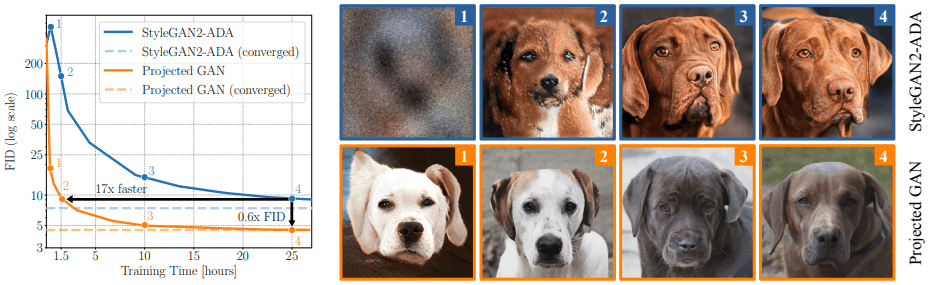## The proposed method (Projected GAN)

GAN consists of a Generator $G$ and a Discriminator $D$.

where $G$ is the latent vector $z$ sampled from a simple distribution (usually normal) $P_z$ to generate the corresponding sample $G(z)$.

Also $D$ is trained to discriminate between real samples $x~P_x$ and generated samples $G(z)~P_{G(z)}$.

In this case, the objective function of GAN is expressed by the following equation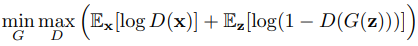In the proposed method, Projected GAN, we introduce a set of feature projectors ($\{P_l\}$) that transforms real and generated images into the input space of the discriminator. In this case, the previously mentioned objective function is replaced by the following equation.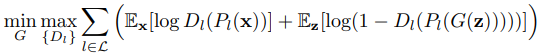Here, $\{D_l\}$ is the set of discriminators corresponding to different feature projectors $P_l$ in $\{P_l\}$. Next, we will discuss more specific configurations of generators, discriminators, and feature projectors.

### Model Overview

Among Projected GANs, the generators are from existing GAN methods (StyleGAN2-ADA, FastGAN, etc.). Therefore, this section focuses on the discriminator $D_l$ and feature projector $P_l$.

### Multi-scale discriminator

As explained in the introduction, Projected GAN uses a representation of a pre-trained model for the discriminator.

Specifically, we obtain features from four layers of the pre-trained network $F$ (with resolutions of $L_1=64^2, L_2=32^2, L_3=16^2, and L_4=8^2$, respectively). We then pass each resolution feature through a feature projector $P_l$ and introduce a simple convolutional architecture as the corresponding discriminator $D_l$. Roughly, the structure is as follows.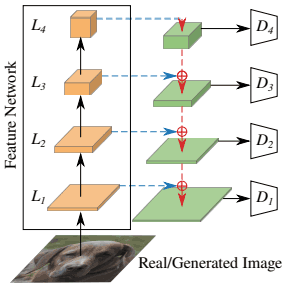As shown in this figure, for each layer representation of the pre-trained model $L_1, L_4$ of the pre-trained model are passed through a feature projector and input to the corresponding discriminator $D_1,..., D_4$. ,D_4$. Also, the discriminators$D_l$are all set to an output resolution of$4x4$(by adjusting the number of downsampling layers), and these logits are summed to compute the overall loss. Also, the architecture of the discriminator is as follows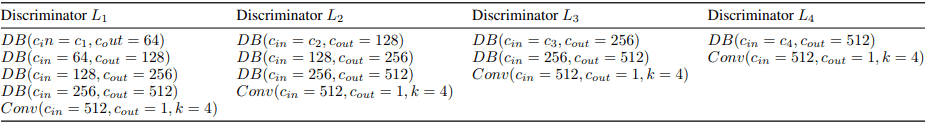Regarding the table, DB(DownBlock) consists of convolution with kernel size 4 and stride 2, BatchNorm, and LeakyReLU (slope 0.2). Also, spectral normalization is applied to all convolution layers. ### Random Feature Projector Next, the feature projector consists of two components: Cross-Channel Mixing (CCM) and Cross-Scale Mixing (CSM). These are random and fixed and are not updated during training (only the Generator and Discriminator are updated during training). CCM(Cross-Channel Mixing) Cross-Channel Mixing (CCM) mixes features at the channel level by performing a$1x1$random convolution operation on features obtained from a pre-trained model. This corresponds to the blue arrow in the following figure.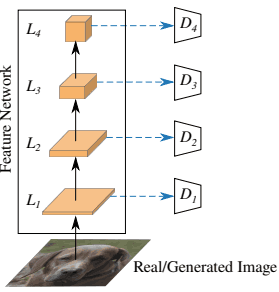The weights of this convolutional layer are randomly initialized by Kaiming initialization. CSM (Cross-Scale Mixing) Cross-Scale Mixing (CSM) consists of processing with a$3x3$convolutional layer and a Bilinear upsampling layer that mixes features of different resolutions, as represented in the following figure.The CSM corresponds to the red arrow in the figure. With the addition of this process, the architecture becomes U-Net-like. The weights are initialized randomly as in CCM. ### On pre-trained models There are various possible pre-trained models for feature representation extraction, but the following models are used in the experiments in the original paper. Of these, EfficientNet(lite1) showed the best results, so EfficientNet(lite1) is used in the absence of any mention. ## experimental results ### Comparison with state-of-the-art models In the experiments, we first present the comparison results with existing state-of-the-art GAN models. Here, we use StyleGAN2-ADA and FastGAN as baselines for comparison. We use FID (Fréchet Inception Distance) as our evaluation metric. (In the appendix of the original paper, the results for different evaluation metrics such as KID, SwAV-FID, precision, and recall are also reported.) Convergence speed and sample efficiency First, we compare the convergence speed and sample efficiency on the LSUN-Church and CLEVR data sets. In this case, the comparison results for convergence speed are as follows.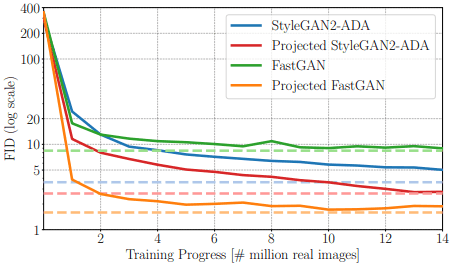Regarding the figure, Projected StyleGAN2-ADA and Projected FastGAN are Projected GANs that use the corresponding architecture as a generator. As shown in the figure, FastGAN converges early, but the FID score saturates at a high value; StyleGAN2-ADA converges slowly, but the FID drops to a low value. The proposed method, Projected GAN, shows a good improvement in both convergence speed and FID, especially when the architecture of FastGAN is used. Surprisingly, the performance of StyleGAN2-ADA trained on 88M images (blue dotted line in the figure) is achieved by Projected FastGAN at 1.1M images, which clearly shows the effectiveness of the proposed method. Because of its high performance, the model adopted as the generator of FastGAN is used as the proposed method in the following, and it is called Projected GAN. Next, the comparison of sample efficiency is as follows.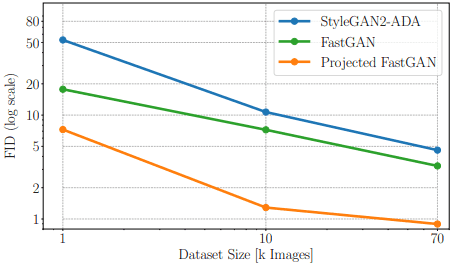As shown in the figure, the performance in the case of small datasets is also shown to be very good compared to the existing methods. Comparison of large and small data sets Next, the comparison results for data sets of various sizes and resolutions are as follows.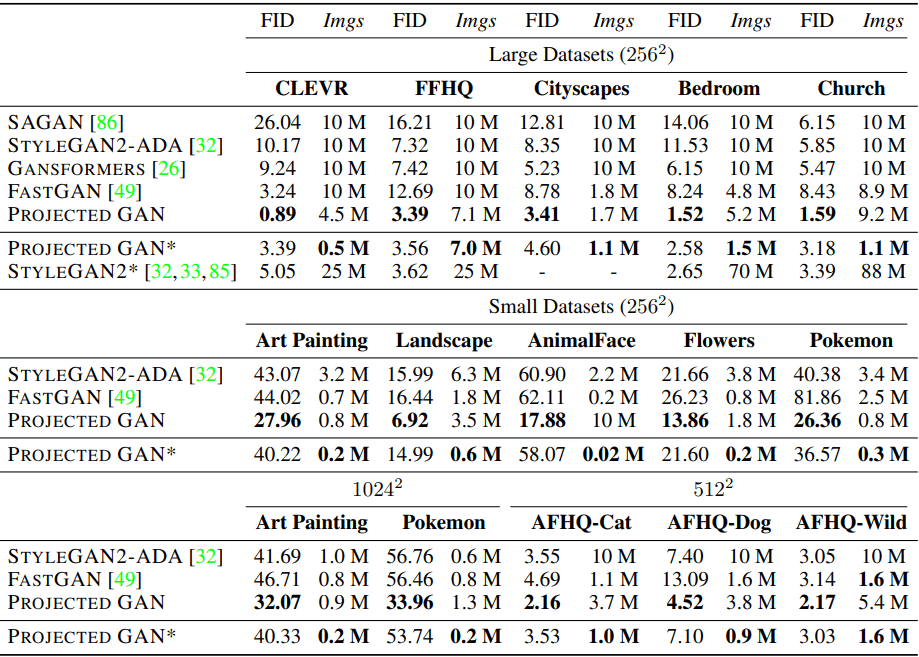In the table, PROJECTED GAN* indicates when the proposed method exceeds the best performance of the existing models. For example, CLEVR has been shown to outperform the lowest FID scores in the previous literature when trained on 0.5M images. As shown in the table, the proposed method significantly outperforms the existing methods in terms of both FID score and data efficiency for all cases of Large/Small Datasets and resolutions ($256^2,512^2,1024^2\$).

In addition, even though EfficientNet trained with ImageNet is consistently used as the pre-trained model, good results are obtained on various data sets. This shows the versatility of the features of the model trained with ImageNet.

### Ablation Research

In the following sections, we will perform ablation studies. Initially, the comparison results of the pre-trained models used as feature extractors are as follows.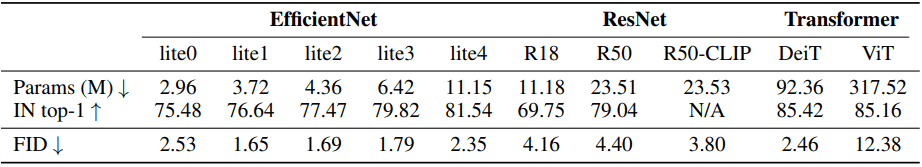As shown in the table, the correlation between ImageNet top-1 accuracy and FID score seems to be small, with EfficientNet-lite1 showing the best results. The ability to achieve high performance on relatively small models is a beneficial feature in reducing the overall computational cost.

In addition, the ablation results for the discriminator and feature projector are as follows.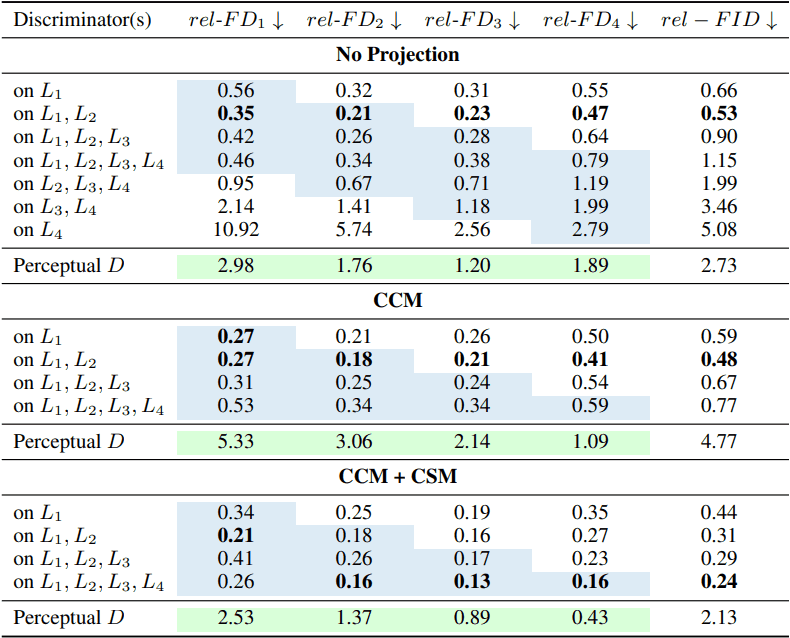In general, it was shown that better results can be obtained by using multi-scale features and by using a feature projector consisting of CCM and CSM.

## summary

Existing state-of-the-art GAN models, such as StyleGAN2-ADA, suffer from challenges such as the huge computational cost of training.

In the paper presented in this article, by using the features of the pre-trained model, we have shown that the quality of the generated images, sample efficiency, and convergence speed all significantly outperform the existing state-of-the-art models.

This result not only achieves better image generation but also enables people without large computational resources to create and study state-of-the-art GAN models. However, the proposed method may not generate the non-face parts well or may generate images with artifacts as shown in the following figure.Despite these issues, the emergence of GAN models with excellent efficiency and quality, such as the proposed method, will lead to more people being involved in the growth of this field (even though the risk of abuse such as deep faking may increase), and it can be said that this is very important and useful research.

The official code is also available, so you may want to check it out along with the original paper.

If you have any suggestions for improvement of the content of the article,